## Signal basics, fft, and aliasing in Matlab

This lab explores basic aspects of sin/cos waves and plotting in Matlab. It then explores the Fourier Transform (FFT) a bit. The FFT is probably the most important transformation in signal processing.
More detailed explanations can be found on Michael X. Cohen's excellent Youtube channel.
Here is a screen shot that shows the relationship between time domain signals (top row) and frequency domain signals (bottom row) for some simple sine waves: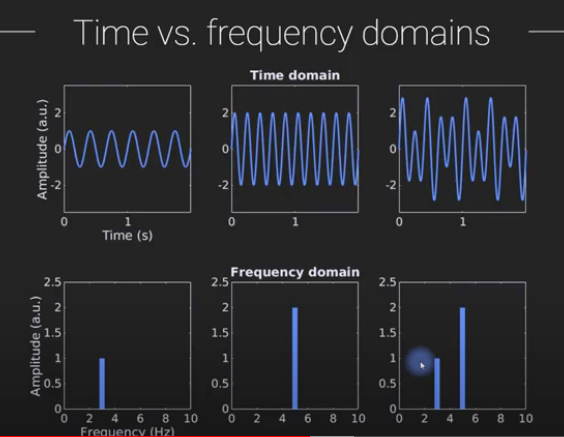### Signal basics

First, let's create a sine wave. The formula for this is: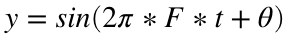Fs = 1000; % Sampling frequency, e.g., Hz (samples/sec)
T = 1/Fs; % Sampling period (time between samples, e.g., sec)
L = 1000; % Length of signal (in samples)
t = (0:L-1)*T; % Time vector
% Sine wave parameters
theta = 0; % In radians
F = 10; % Frequency of sin wave (cycles/sec)
y = sin(2 * pi * F * t + theta);
create_figure('sin wave');
plot(t, y)
xlabel('Time (sec)')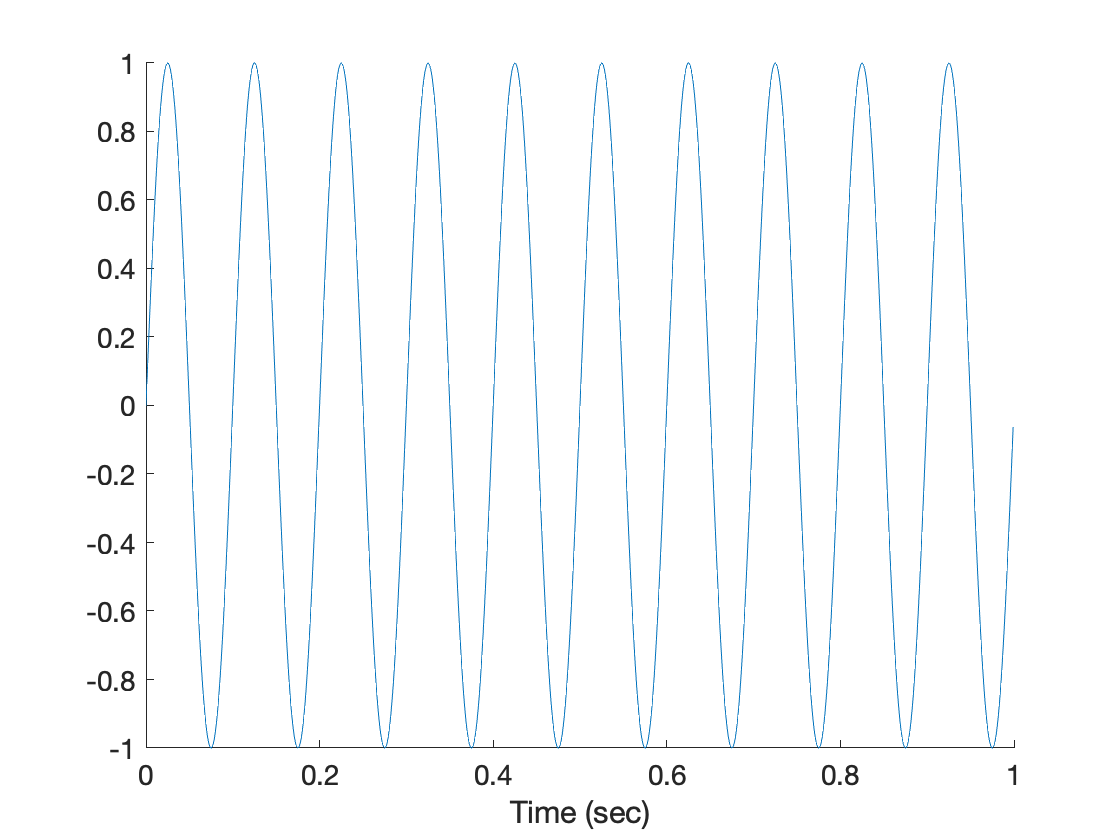Try changing F to 20. What would you expect to happen? What happens?
Theta (θ) is often used to reference an angle, as it is here. θ in the equation above is the offset, or phase shift. The other part of the equation,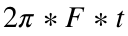, provides the angle based on frequency (F) and time (t).
Try changing theta, θ. What happens with positive θ? Negative θ?
θ is specified in radians, with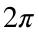radians equal to 360 degrees (a full circle), or πequal to 180 degrees, a semicircle.
Try replacing sin with cos above, to generate a cosine wave. What is the value of cos(θ), where theta is the angle, at time 0 (0 radians?)
Try typing sin(0) and cos(0).
What theta would shift the sin wave by 90 degrees, so it looks like the cosine wave?

### The FFT and aliasing

The function fft( ) estimates the coefficients of the Fourier transform, transforming a time-domain signal (i.e., an observed fMRI time series) into a series of sine waves with different amplitudes and phases.
Fourier coefficients are complex numbers, with values in the real and imaginary planes. If you are intrigued, check out this video for a great walk-through. The magnitude of this signal is what we're mainly interested in plotting here, and it relates to the power at a particular frequency. That's the length of the coefficient in complex space, as shown in the image below, and is extracted using abs(yf), where yf are the estimated Fourier coefficients. The phase is the angle of the vector as it moves through the complex plane.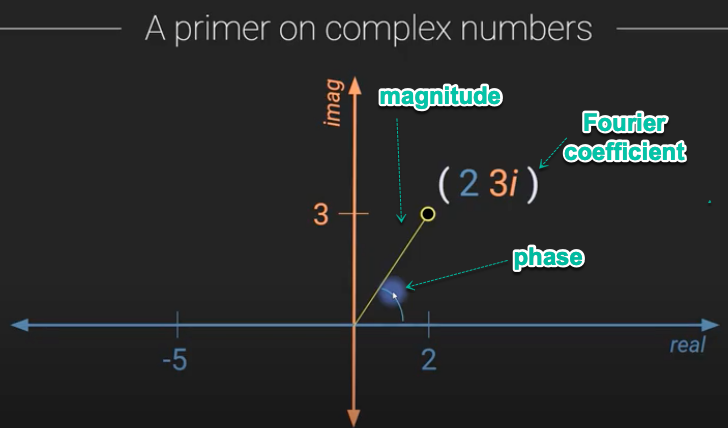We can think of sine waves as projections onto one axis of a vector traveling around a circle, like the hand of a clock. If we're looking at the position of the clock hand only on one axis, it will make a sine wave-type pattern. In the case of the Fourier transform, the two dimensions are the real and imaginary number lines. We can think of Fourier coefficients describing a 3-d sine wave that spirals through time (t) with frequency f. The higher the frequency, the tighter the spiral. The larger the magnitude, the larger the spiral's radius is (its path is farther from the origin).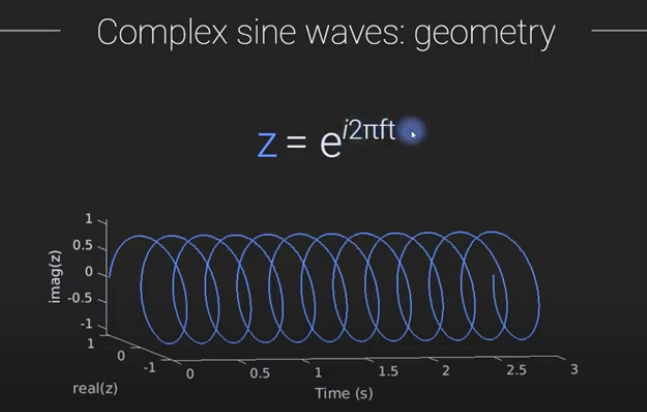Image credits: Michael X. Cohen
create_figure('sin wave', 1, 2);
plot(t, y)
xlabel('Time (sec)')
subplot(1, 2, 2)
yf = fft(y); % Fourier transform
yf = abs(yf/L); % When X is complex, ABS(X) is the complex modulus (magnitude) of the elements of X
f = Fs*(0:(L-1))/L; % define frequencies for each element of yf
plot(f, yf)
nyquist = Fs ./ 2; % Any signal faster than Nyquist freq will be aliased
h = plot_vertical_line(nyquist);
set(h, 'LineStyle', ':')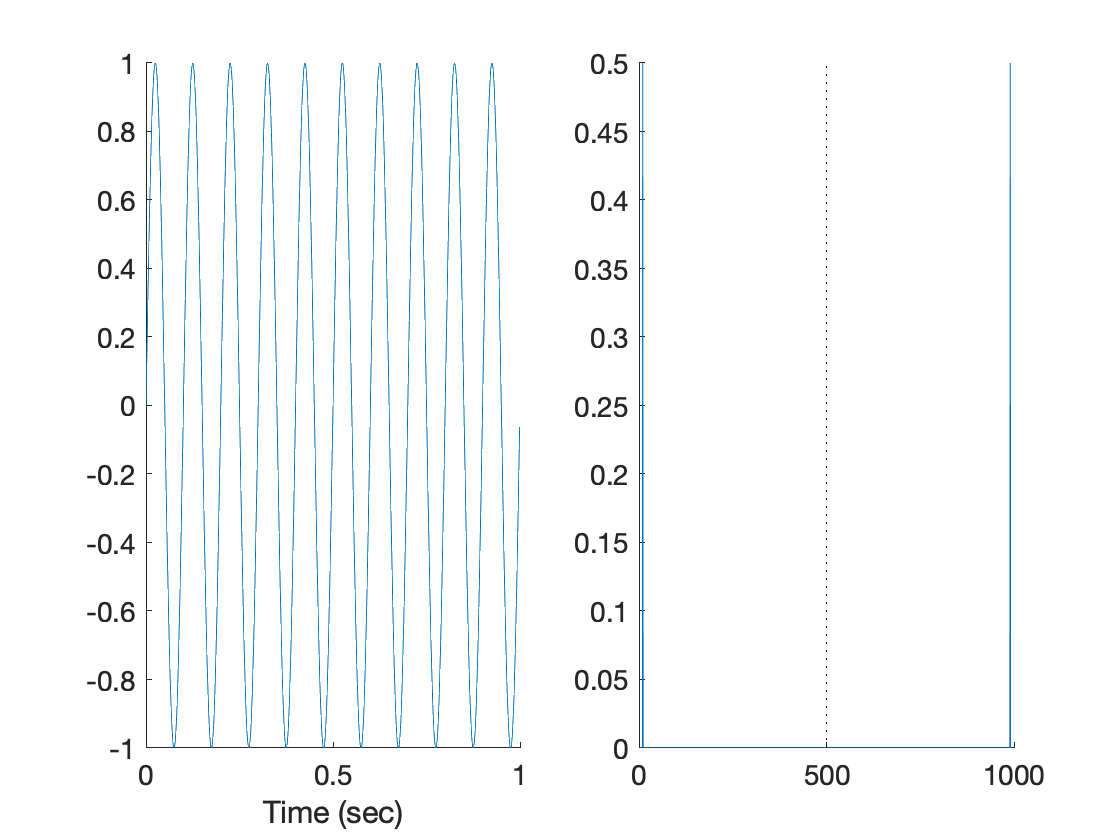The Nyquist frequency is 1/2 the sampling rate. Any signal with frequencies higher than that (faster oscillation) will be aliased back into the low frequency range.
In these examples, the sampling frequency is very high (e.g., 1000 Hz), and the underlying frequency of the sin wave is very low. So there is no problem with aliasing. Now let's look at a case where we're sampling a faster-moving underlying frequency with a lower sampling rate.
Fs = 50; % Rate we're sampling at: Sampling frequency, e.g., Hz (samples/sec)
T = 1/Fs; % Sampling period (time between samples, e.g., sec)
L = 150; % Length of signal (in samples)
t = (0:L-1)*T; % Time vector
% Sine wave parameters
theta = 0; % In radians
F = 10; % Frequency of underlying signal we're detecting (cycles/sec, e.g., Hz)
y = sin(2 * pi * F * t + theta);
create_figure('sin wave', 1, 2)
ans =
Figure (sin wave) with properties: Number: 2 Name: 'sin wave' Color: [1 1 1] Position: [617 599 560 420] Units: 'pixels' Show all properties
subplot(1, 2, 1)
plot(t, y)
xlabel('Time (sec)')
title('Time domain')
subplot(1, 2, 2)
yf = fft(y); % Fourier transform
yf = abs(yf/L); % When X is complex, ABS(X) is the complex modulus (magnitude) of the elements of X
yf = 2 * yf(1:L/2+1); % Take only the half of frequencies below the nyquist
f = Fs*(0:(L/2))/L; % define frequencies for each element of yf
plot(f, yf)
xlabel('Frequency (Hz)')
title('Frequency domain')
nyquist = Fs ./ 2; % Any signal faster than Nyquist freq will be aliased
h = plot_vertical_line(nyquist);
set(h, 'LineStyle', ':')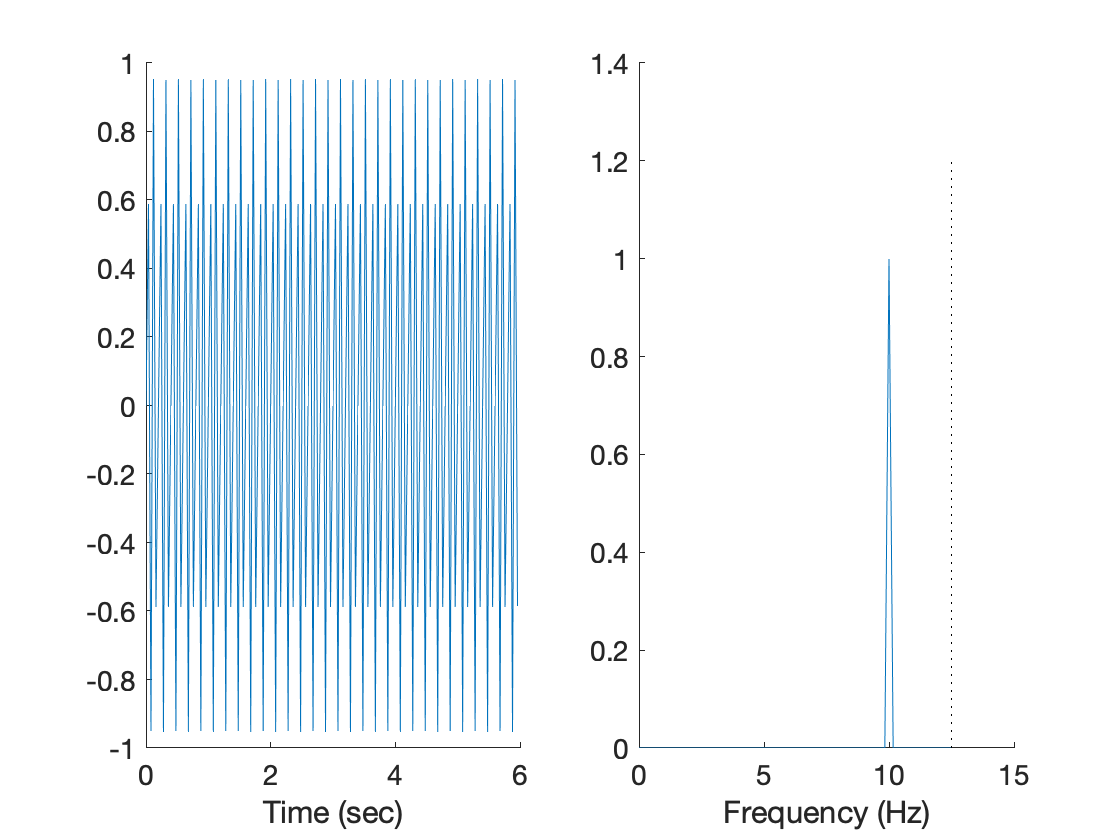This is 50 Hz sampling for a 10 Hz signal. The Nyquist frequency is the dashed line. The sampling rate is 5x higher, so we're cool. What is the frequency of this sin wave? Does it match what we put in when we created the signal (Fs)?
What signal frequencies (Fs) would you expect to become problematic based on the Nyquist frequency? 10, 15, 20, 25, 30, 40...?
What happens when you go up to Fs = 20? What happens when Fs is at the Nyquist frequency of 25? What happens as you go further above that?
At what sampling frequency does the ability to detect a Fs = 10 Hz signal break down? 40...30...25...20...10...?